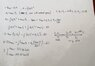# Motion in one dimension

• hquang001
Yes i have calculated all the quantities you mentioned, and find each distance x1,x2,x3 which add up 60km. So i think this should be correct. Thank you very much for your help!

#### hquang001

Homework Statement
A train which connects city A to C, leaves the station A at 10:06 AM. The train accelerates for 6 minutes with a constant rate before it reaches its top speed. Then it is travelling with constant speed. Then it reduces its speed with a constant rate of 600 km/h² before it reaches the station of city C (The value of the speed is 0km/h when it reaches the station C). The train should arrive at city C at 10:42 AM. The distance between A to C is 60km

a) What is the acceleration of the train in the beginning ?
b) What is the maximum speed of the train ?
Relevant Equations
X = Xo + Vot + 1/2 at²
V = Vo + at
V² - Vo² = 2aX
total time: t = 36 mins = 0.6h = t1 + t2
=> t2 = t - t1 = 0.6h - 0.1h = 0.5h

Vmax = a1 x t1
Vat C = Vmax + a2t2
substitute Vmax in Vat C we have : 0 = a1 x 0.1h + (-600 km/h²) x 0.5h => a1 = 3000km/h²
Vmax = a1 x t1 = 3000 x 0.1 = 300km/h

I check the result by:
x1 = ½ a
1 t1² = ½ . (3000). (0.1²) = 15km
x = ½ a
2t2² + vmaxt2 + x1 = ½ (-600).(0.5²) + (300).(0.5) + 15km = 90km

I don't know why the total distance when i check the result turn out to be 90km, while the questions states that AC is 60km

hquang001 said:
(-600 km/h²) x 0.5h
It is not slowing down for half an hour. It spends some unstated time at constant speed.

@hquang001 If I were you, I'd draw a speed vs time graph, where the distance is the area under the graph. It's easy to get lost if you only use the SUVAT equations.

haruspex said:
It is not slowing down for half an hour. It spends some unstated time at constant speed.
This is the hint which i forgot to put in the question statement: It could be that you get more than one answer .

After considering your help and calculating again, i really got two possible maximum speed value like the hint statedI want to ask how i can check if my results are correct in situation like this, or in general, one dimensional motion ?

Last edited:
PeroK said:
@hquang001 If I were you, I'd draw a speed vs time graph, where the distance is the area under the graph. It's easy to get lost if you only use the SUVAT equations.
I have done it again and also include a simple graph

hquang001 said:
This is the hint which i forgot to put in the question statement: It could be that you get more than one answer .

After considering your help and calculating again, i really got two possible maximum speed value like the hint stated View attachment 278640

Have you checked your solutions? Do they both work out?

PeroK said:
Have you checked your solutions? Do they both work out?
Only one solution is possible
When i substitute V = 522.09 km/h to find t3,
t3 = 0.87h which will make t2 negative

hquang001 said:
Only one solution is possible
When i substitute V = 522.09 km/h to find t3,
t3 = 0.87h which will make t2 negative
Okay, so you have one good solution?

PeroK said:
Okay, so you have one good solution?
Yes, Vmax = 137.91 is possible
but i don't know if it's correct or not, i don't know how to check it

hquang001 said:
Yes, Vmax = 137.91 is possible
but i don't know if it's correct or not, i don't know how to check it
You have to calculate the other quantities: initial acceleration; ##t_3##, ##t_2## and check you get the right time and distance for the journey. Tricky question!

PeroK said:
You have to calculate the other quantities: initial acceleration; ##t_3##, ##t_2## and check you get the right time and distance for the journey. Tricky question!
Yes i have calculated all the quantities you mentioned, and find each distance x1,x2,x3 which add up 60km. So i think this should be correct. Thank you very much for your help

•PeroK